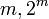# Groups of order 2^m.3^n

This article discusses groups whose order has prime factors in the set$\{ 2, 3 \}$ and no others. Another way of putting this is that the article discusses finite$\pi$-groups where$\pi = \{ 2, 3\}$.

There are only two prime factors of this number. Order has only two prime factors implies solvable (by Burnside's$p^aq^b$-theorem) and hence all groups of this order are solvable groups (specifically, finite solvable groups). Another way of putting this is that the order is a solvability-forcing number. In particular, there is no simple non-abelian group of this order.

## Orders of interest

The clickable links in the table are to pages devoted to groups of that order.

The rows represent values of$m$ and corresponding values of$2^m$. The columns represent values of$n$ and corresponding values of$3^n$.$m$$2^m$ generic$n$$n = 0$$n = 1$$n = 2$$n = 3$$n = 4$$n = 5$$n = 6$$n = 7$.
-- --$3^n = 1$$3^n = 3$$3^n = 9$$3^n = 27$$3^n = 81$$3^n = 243$$3^n = 729$$3^n = 2187$
generic generic 2^m 3.2^m 3^2.2^m 3^3.2^m 3^4.2^m 3^5.2^m 3^6.2^m 3^7.2^m
0 1 3^n 1 3 9 27 81 243 729 2187
1 2 2.3^n 2 6 18 54 162 486 1458 4374
2 4 2^2.3^n 4 12 36 108 324 972 2916 8748
3 8 2^3.3^n 8 24 72 216 648 1944 5832
4 16 2^4.3^n 16 48 144 432 1296 3888
5 32 2^5.3^n 32 96 288 864
6 64 2^6.3^n 64 192 576 1728
7 128 2^7.3^n 128 384 1152
8 256 2^8.3^n 256 768
9 512 2^9.3^n 512 1536
10 1024 2^10.3^n 1024

## Counts of groups

### Counts of all groups

The rows give values of$m, 2^m$ and the columns give values of$n, 2^n$. Each cell value is the total number of isomorphism classes of groups of order$2^m \cdot 3^n$.

Note that for small values of$m,n$, the order of magnitude of the values depends roughly on$m + n$ (so it is roughly of the same order of magnitude as we move along a north-east-to-south-west diagonal). This fails to hold for larger values of$m,n$.$m$$2^m$$n = 0$$n = 1$$n = 2$$n = 3$$n = 4$$n = 5$$n = 6$$n = 7$.
-- --$3^n = 1$$3^n = 3$$3^n = 9$$3^n = 27$$3^n = 81$$3^n = 243$$3^n = 729$$3^n = 2187$
0 1 1 1 2 5 15 67 504 9310
1 2 1 2 5 15 55 261 1798
2 4 2 5 14 45 176 900
3 8 5 15 50 177 757 3973
4 16 14 52 197 775 3609
5 32 51 231 1045 4725
6 64 267 1543 8681 47937
7 128 2328 20169 157877
8 256 56092 1090235
9 512 10494213 408641062
10 1024 49487365422

### Counts of nilpotent groups

Thus, the number of nilpotent groups of order$2^m \cdot 3^n$ = (number of groups of order$2^m$)$\times$ (number of groups of order$3^n$)$m$$2^m$$n = 0$$n = 1$$n = 2$$n = 3$$n = 4$$n = 5$$n = 6$$n = 7$.
-- --$3^n = 1$$3^n = 3$$3^n = 9$$3^n = 27$$3^n = 81$$3^n = 243$$3^n = 729$$3^n = 2187$
0 1 1 1 2 5 15 67 504 9310
1 2 1 1 2 5 15 67 504 9310
2 4 2 2 4 10 30 134 1008 18620
3 8 5 5 10 25 75 335 2520 46550
4 16 14 14 28 70 210 938 7056 130340
5 32 51 51 102 255 765 3417 25704 474810
6 64 267 267 534 1335 4005 17889 134568 2485770
7 128 2328
8 256 56092
9 512 10494213
10 1024 49487365422